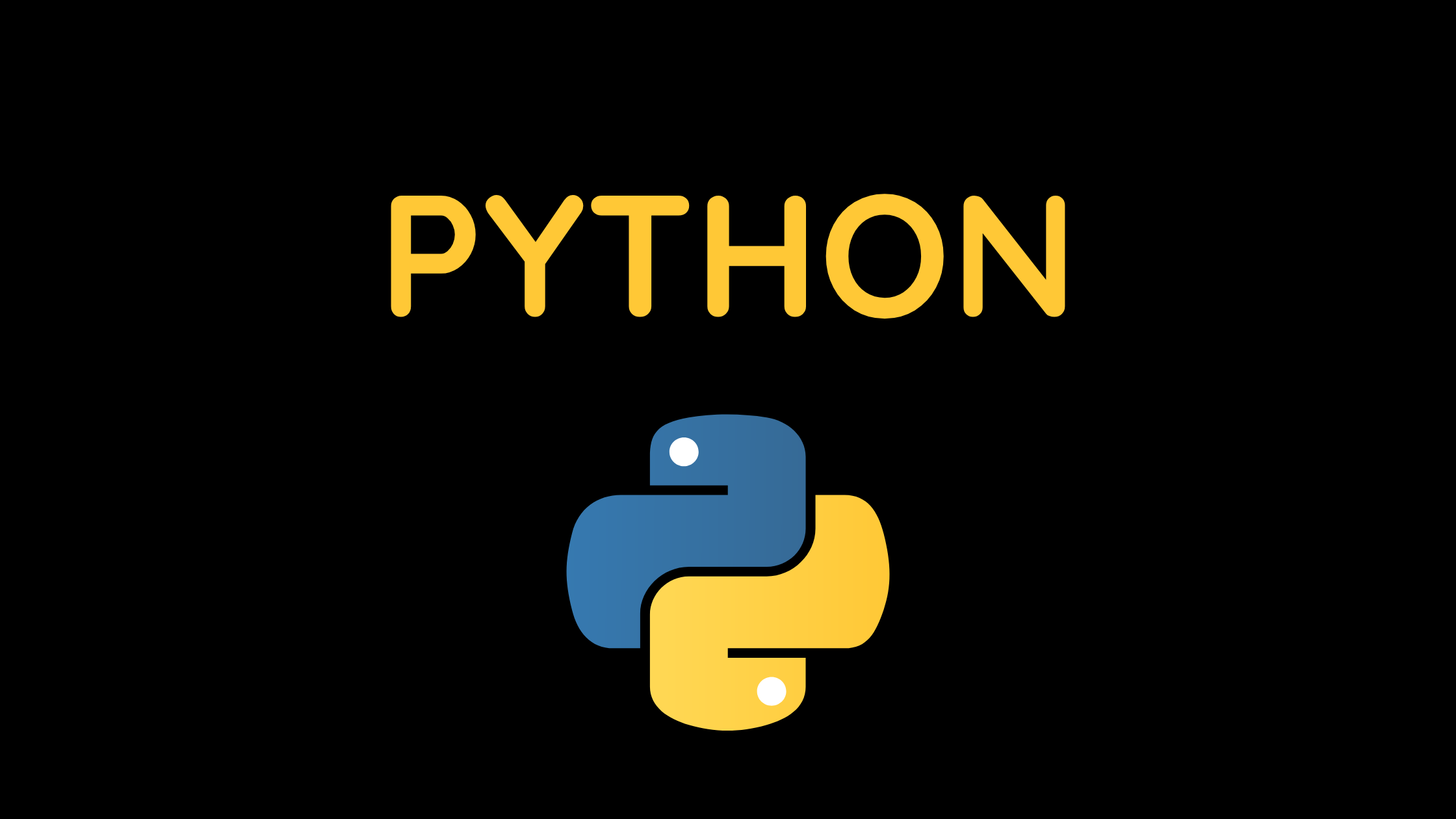## Looping Constructs in Python: A Guide to for and while Loops

Welcome to our comprehensive guide on looping constructs in Python. Looping constructs, such as the for loop and while loop, are essential tools for performing repetitive tasks and iterating over data in your Python programs. In this guide, we will explore these looping constructs and provide examples to help you understand their usage.

Contents

## Understanding the for Loop in Looping constructs

The for loop is used to iterate over a sequence (such as a list, tuple, or string) or other iterable objects in Python. It allows you to execute a block of code repeatedly for each item in the sequence.

```for item in sequence:
# Code to execute for each item```

Example: Iterating over a list using a for loop

```fruits = ["apple", "banana", "cherry"]

for fruit in fruits:
print(fruit)```

## Working with the while Loop In Looping constructs

The while loop is used to repeatedly execute a block of code as long as a given condition is true. Unlike the for loop, the while loop’s condition is evaluated before each iteration.

```while condition:
# Code to execute while the condition is true```

Example: Performing a countdown using a while loop

```count = 5

while count &gt; 0:
print(count)
count -= 1```

## Nested Loops In Looping constructs

Python allows you to nest loops within one another, enabling you to perform more complex iterations. This is particularly useful when dealing with multi-dimensional data structures or when you need to perform operations on multiple levels of a hierarchy.

Example: Printing a pattern using nested loops

```for i in range(1, 6):
for j in range(1, i + 1):
print("*", end=" ")
print()```

Output:

```*
* *
* * *
* * * *
* * * * *```

## Loop Control Statements In Looping constructs

Python provides loop control statements, such as `break` and `continue`, to modify the behavior of loops. The `break` statement allows you to exit a loop prematurely, while the `continue` statement skips the current iteration and moves to the next one. These statements give you greater control over the flow of your loops.

Example: Finding a number in a list using break

```numbers = [10, 20, 30, 40, 50]

for num in numbers:
if num == 30:
print("Number found!")
break
else:

Output:

`Number found!`

## Iterating with Range In Looping constructs

The `range()` function in Python generates a sequence of numbers that can be used with looping constructs. It is often used in conjunction with the for loop to iterate a specific number of times or over a range of values.

Example: Summing numbers using range and for loop

```total = 0

for i in range(1, 11):
total += i

print("Sum:", total)```

Output:

`Sum: 55`

## Infinite Loops

While loops can be used to create infinite loops by setting the condition to `True`. However, caution must be exercised to include a terminating condition or a proper exit strategy within the loop to avoid an endless execution.

Example: Creating an infinite loop with user input

```while True:
name = input("Enter your name (type 'exit' to quit): ")
if name == 'exit':
break
print("Hello,", name)```

## Optimizing Loop Performance

When working with large data sets or performing computationally intensive operations, optimizing loop performance becomes crucial. Techniques such as list comprehension and using built-in functions like `map()` and `filter()` can help improve the efficiency of your loops.

Example: Squaring numbers using list comprehension

```numbers = [1, 2, 3, 4, 5]
squared = [num**2 for num in numbers]
print(squared)```

Output:

```[1, 4, 9, 16, 25]
```

These examples showcase the practical application of nested loops, loop control statements, iterating with range, infinite loops, and optimizing loop performance. Incorporating these techniques into your Python code will enhance its functionality and efficiency.

## Conclusion

By mastering looping constructs in Python, including the for loop and while loop, and understanding concepts like nested loops, loop control statements, iterating with range, infinite loops, and optimizing loop performance, you gain a powerful set of tools to handle repetitive tasks, iterate over data, and solve complex programming challenges.

Experiment with these looping techniques, explore additional resources, and practice implementing them in your own projects to solidify your understanding. With time and experience, you’ll become more proficient in leveraging looping constructs to write efficient and effective Python code.